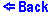Image Formation by a Diverging Lens
You can move the object around by either clicking and draging or just clicking in the location of your choice. A diverging lens always forms an upright virtual image. The image appeares on the left of the lens as a gray arrow.
The above applet shows: two arrows, a diverging lens, and rays of light being emmitted by the red arrow. The red arrow is the object, while the gray arrow is the virtual image that results after the rays have passed through the lens. The applet also displays two focus shown as blue dots.

The image formed by a diverging lens can be made using only three principal rays:

• Ray 1 is the ray which travels parallel to the axis and after going through the lens it passes through the focal point.
• Ray 2 passes through the center of the lens.
• Ray 3 goes through the focal point and then travels parallel to the axis after passing through the lens.
• However, unlike in the converging lens case, the rays do not intersect. Instead the mind assumes the image to be at an imaginary point which is derived by extending the rays and then taking the intersection of the extentions. The extensions are shown in green. Thus any point on the object can be mapped, using the rays above, into a corresponding point on the image.

The above is a useful tecnique, but it usually involves a drawing of some sort. A more practical way is not as complete but is much simplier. You can find the distance of the image from the lens by the following equation:

1/di + 1/do = 1/f,
where di is the distance from the lens to the image, do is the distance from the object to the lens, and f is the focal distance of the lens.
Note that for the diverging mirror f should be negative. This ensures that di is negative and that the image is on the left of the lens (a virtual image), this is the only possibility for a diverging lens.